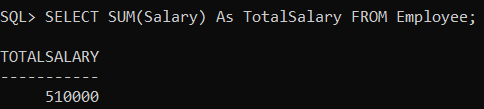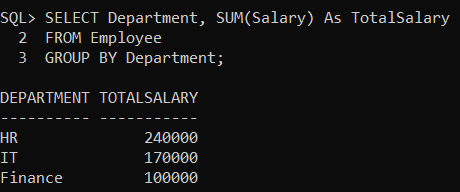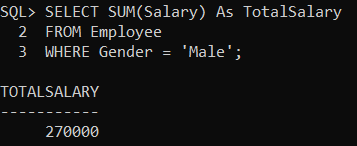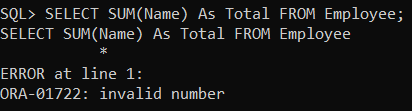# SUM Function in Oracle

## SUM Function in Oracle with Examples

In this article, I am going to discuss the SUM Function in Oracle with Examples. Please read our previous article where we discussed COUNT Function in Oracle with Examples.

##### SUM Function in Oracle:

The SUM Function in Oracle is used to return the total sum of a given numeric column. The SUM function will only work on numeric data types. For columns containing other than numeric values, it will give you an error i.e. Invalid Number. Following is the syntax to use the SUM Function in Oracle.

SELECT SUM(column) FROM TableName;

##### Examples to Understand SUM Function in Oracle:

We are going to use the following Employee table to understand the need and use of SUM Function in Oracle with Examples.Please execute the below SQL query to drop the existing Employee table and create a new Employee table with the required sample data.

```DROP Table Employee;

CREATE TABLE Employee (
Id INT PRIMARY KEY,
Name VARCHAR(15),
Department VARCHAR(10),
Salary NUMBER(8, 2),
Gender VARCHAR(10),
Age INT,
City VARCHAR(10)
);

INSERT INTO Employee (Id, Name, Department, Salary, Gender, Age, City) VALUES (1001, 'John', 'IT', 35000, 'Male', 25, 'London');
INSERT INTO Employee (Id, Name, Department, Salary, Gender, Age, City) VALUES (1002, 'Smith', 'HR', 45000, 'Female', 27, 'Mumbai');
INSERT INTO Employee (Id, Name, Department, Salary, Gender, Age, City) VALUES (1003, 'James', 'Finance', 50000, 'Male', 28, 'Delhi');
INSERT INTO Employee (Id, Name, Department, Salary, Gender, Age, City) VALUES (1004, 'Mike', 'Finance', 50000, 'Male', 28, 'London');
INSERT INTO Employee (Id, Name, Department, Salary, Gender, Age, City) VALUES (1005, 'Linda', 'HR', 75000, 'Female', 26, 'Mumbai');
INSERT INTO Employee (Id, Name, Department, Salary, Gender, Age, City) VALUES (1006, 'Anurag', 'IT', 35000, 'Male', 25, 'London');
INSERT INTO Employee (Id, Name, Department, Salary, Gender, Age, City) VALUES (1007, 'Priyanla', 'HR', 45000, 'Female', 27, 'Mumbai');
INSERT INTO Employee (Id, Name, Department, Salary, Gender, Age, City) VALUES (1008, 'Sambit', 'IT', 50000, 'Male', 28, 'London');
INSERT INTO Employee (Id, Name, Department, Salary, Gender, Age, City) VALUES (1009, 'Pranaya', 'IT', 50000, 'Male', 28, 'London');
INSERT INTO Employee (Id, Name, Department, Salary, Gender, Age, City) VALUES (1010, 'Hina', 'HR', 75000, 'Female', 26, 'Mumbai');

```
##### SUM Function with Numeric Data Type in Oracle

In our Employee table, the Id, Salary, and Age columns have numeric data types. Let us calculate the Total Salary of all employees. To do so, we need to use the Oracle SUM aggregate function and also we need to pass the Salary as a parameter to the SUM Function as shown in the below SQL Query.

SELECT SUM(Salary) As TotalSalary FROM Employee;

Now when you execute the above query, you will get the following output.##### SUM Function with Group by Clause in Oracle

Now we will calculate the Total Salary of each department from the Employee table. To do so, we need to pass the Salary column to the SUM function as well as we need to group the record by department using the GROUP BY clause as shown in the below SQL Query.

```SELECT Department, SUM(Salary) As TotalSalary
FROM Employee
GROUP BY Department;```

Now when you execute the above SQL query, you will get the following output.##### SUM Function with WHERE Clause in Oracle

Now we need to calculate the total salary of all Male Employees present in the Employee table. To do so, we need to pass the Salary column as a parameter to the SUM function and filter the records by using the WHERE clause as shown in the below SQL Query.

```SELECT SUM(Salary) As TotalSalary
FROM Employee
WHERE Gender = 'Male';```

Now when you execute the above SQL query, you will get the following output.##### SUM Function with String Values in Oracle

If we pass the string values to the SUM function in oracle, then we will get an error i.e. Invalid Number. In the below query, we are passing the Name column to the SUM function.

SELECT SUM(Name) As Total FROM Employee;

Now when you execute the above SQL query, you will get the following error.In the next article, I am going to discuss MIN Function in Oracle with Examples. Here, in this article, I try to explain the SUM Function in Oracle with Examples and I hope you enjoy this SUM Function in Oracle with Examples article.